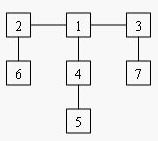Online JudgeProblem SetAuthorsOnline ContestsUser
Web Board
F.A.Qs
Statistical Charts
Problems
Submit Problem
Online Status
Prob.ID:
Register
Authors ranklist
Current Contest
Past Contests
Scheduled Contests
Award Contest
Register
Language:
Balancing Act
 Time Limit: 1000MS Memory Limit: 65536K Total Submissions: 25306 Accepted: 10146

Description

Consider a tree T with N (1 <= N <= 20,000) nodes numbered 1...N. Deleting any node from the tree yields a forest: a collection of one or more trees. Define the balance of a node to be the size of the largest tree in the forest T created by deleting that node from T.
For example, consider the tree:Deleting node 4 yields two trees whose member nodes are {5} and {1,2,3,6,7}. The larger of these two trees has five nodes, thus the balance of node 4 is five. Deleting node 1 yields a forest of three trees of equal size: {2,6}, {3,7}, and {4,5}. Each of these trees has two nodes, so the balance of node 1 is two.

For each input tree, calculate the node that has the minimum balance. If multiple nodes have equal balance, output the one with the lowest number.

Input

The first line of input contains a single integer t (1 <= t <= 20), the number of test cases. The first line of each test case contains an integer N (1 <= N <= 20,000), the number of congruence. The next N-1 lines each contains two space-separated node numbers that are the endpoints of an edge in the tree. No edge will be listed twice, and all edges will be listed.

Output

For each test case, print a line containing two integers, the number of the node with minimum balance and the balance of that node.

Sample Input

```1
7
2 6
1 2
1 4
4 5
3 7
3 1
```

Sample Output

`1 2`

Source

[Submit]   [Go Back]   [Status]   [Discuss]Home PageGo BackTo top